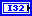# Digital Demod:M Property

RFmx Demod 19.1 Help

Edition Date: October 2019

Part Number: 374641M-01

»View Product Info

### Download Help (Windows Only)RFmx Demod 19.1 HelpRFmx Demod 20.0 HelpRFmx Demod 20.6 Help

Short Name: DDemod M

Property of RFmxDemod

Specifies the M-ary number, which is the number of distinct states that represent symbols in the complex baseband modulated waveform.

The M-ary number is calculated using the following formula:

M = 2^(bits per symbol)

Recommended values of M for the modulation types are as follows:

You do not need to use a selector string to configure or read this property for the default signal instance. Refer to the Selector Strings topic for information about the string syntax for named signals.

The default value is 4.

 2 (2) The M-ary number is 2. 4 (4) The M-ary number is 4. 8 (8) The M-ary number is 8. 16 (16) The M-ary number is 16. 32 (32) The M-ary number is 32. 64 (64) The M-ary number is 64. 128 (128) The M-ary number is 128. 256 (256) The M-ary number is 256. 512 (512) The M-ary number is 512. 1024 (1024) The M-ary number is 1,024. 2048 (2048) The M-ary number is 2,048. 4096 (4096) The M-ary number is 4,096.

## Remarks

The following table lists the characteristics of this property.

 DatatypePermissions Read/Write High-level VIs RFmxDemod DDemod Configure M, RFmxDemod DDemod Configure Modulation Type Resettable Yes

Not Helpful